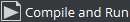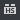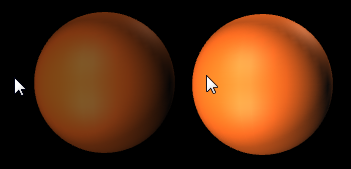## Viz Artist User Guide

Version 5.0 | Published December 20, 2022 ©

# Create and Run Scripts

This section contains two script examples:

• Create and Run Scripts: This example shows how to write a simple script that controls the alpha value of an object.

• Example II: This example shows how to write a simple script that scales the x-scaling value of a container, based on x-scaling value of another container.

# Example I

1. Add a Sphere to a Container.

2. Add a Material and some light.

3. Create a script object.

1. Drag the built-in Script plug-in from the Container plug-ins’ Global folder onto a Container.

2. Click on the Script plug-in icon in a Container

3. Copy and paste the code, below, into the Script Editor.

`Sub OnEnter()`
` Alpha.Value = ``100.0`` `
`End Sub `
`Sub OnLeave()`
` Alpha.Value = ``50.0`` `
`End Sub`

This script consists of two event procedures, OnEnter and OnLeave, which are recognized by the system and invoked whenever the cursor enters or leaves the area occupied by the container in the output window. Take a closer look at what happens inside OnEnter:

`Alpha.Value = ``100.0`

This code sets the alpha value of the container to 100 (the maximum value). If there is no Alpha object, no action occurs, so make sure that the container has an alpha object by adding the Alpha plug-in. Alpha refers to the container’s alpha object, Value is a member (in this case: the only member) of the alpha object, representing its value. Changes made to Alpha.Value are immediately made visible in the output window.
Similarly, the code in the body of OnLeave sets the alpha value back to 50.

4. Add the Alpha plug-in to the container.

5. Execute the script, click.

6. Click Edit to return to editing mode if required. To interact with the scene click thebutton (Scene Editor Buttons) in the Scene Editor.

Note: Thebutton offers a good way for designers to test touch-based interaction points added to a scene. The script is then the logic which triggers what happens next (i.e. events).# Example II

This example defines the event procedure OnExecPerField, which is called for each field. The functions and amount of code used in this procedure needs to be decided upon with great consideration. Include only the code that requires execution on a per-field basis, to keep the resulting performance impact as low as possible.`Dim cube As Container = Scene.FindContainer(“Circle”)`
`Sub OnExecPerField()`
` If scaling.x > ``1.5`` Then`
` cube.Scaling.x = ``2.0`
` Else`
` cube.Scaling.x = ``1.0`
` End If `
`End Sub`

In the first line, a variable named cube, of type Container, is defined:

`Dim cube As Container = Scene.FindContainer(“Cube”)`

Variables of type Container can be initialized to point to a container in the scene tree. If there is no such container, cube is set to Null, the consequence being that any further operations on cube have no effect.
The reason why this is done on a global space is that if you called it inside the OnExecPerField, this code would be executed on every single field, impacting performance.

Now, check if the x-scaling of the current container (that is, the container holding the script) is greater than 1.5, and, depending on the result, set the x-scaling of the container named cube to 2.0 or 1.0:

`If Scaling.x > ``1.5`` Then`
` cube.Scaling.x = ``2.0`` `
`Else`
` cube.Scaling.x = ``1.0`` `
`End If`

Note: The expression Scaling.x refers to the current container, while cube.Scaling.x refers to the container referenced by the variable cube.